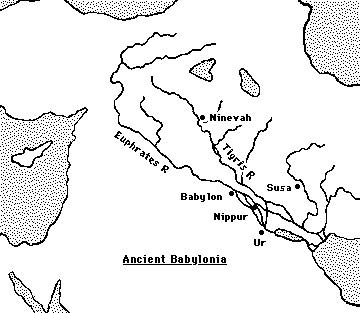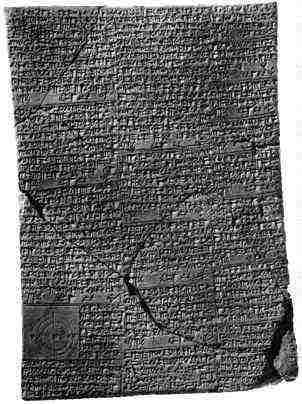# An overview of Babylonian mathematics

The Babylonians lived in Mesopotamia, a fertile plain between the Tigris and Euphrates rivers.Here is a map of the region where the civilisation flourished.

The region had been the centre of the Sumerian civilisation which flourished before 3500 BC. This was an advanced civilisation building cities and supporting the people with irrigation systems, a legal system, administration, and even a postal service. Writing developed and counting was based on a sexagesimal system, that is to say base 60. Around 2300 BC the Akkadians invaded the area and for some time the more backward culture of the Akkadians mixed with the more advanced culture of the Sumerians. The Akkadians invented the abacus as a tool for counting and they developed somewhat clumsy methods of arithmetic with addition, subtraction, multiplication and division all playing a part. The Sumerians, however, revolted against Akkadian rule and by 2100 BC they were back in control.

However the Babylonian civilisation, whose mathematics is the subject of this article, replaced that of the Sumerians from around 2000 BC The Babylonians were a Semitic people who invaded Mesopotamia defeating the Sumerians and by about 1900 BC establishing their capital at Babylon.

The Sumerians had developed an abstract form of writing based on cuneiform (i.e. wedge-shaped) symbols. Their symbols were written on wet clay tablets which were baked in the hot sun and many thousands of these tablets have survived to this day. It was the use of a stylus on a clay medium that led to the use of cuneiform symbols since curved lines could not be drawn. The later Babylonians adopted the same style of cuneiform writing on clay tablets.Here is one of their tablets

Many of the tablets concern topics which, although not containing deep mathematics, nevertheless are fascinating. For example we mentioned above the irrigation systems of the early civilisations in Mesopotamia. These are discussed in  where Muroi writes:-
It was an important task for the rulers of Mesopotamia to dig canals and to maintain them, because canals were not only necessary for irrigation but also useful for the transport of goods and armies. The rulers or high government officials must have ordered Babylonian mathematicians to calculate the number of workers and days necessary for the building of a canal, and to calculate the total expenses of wages of the workers.

There are several Old Babylonian mathematical texts in which various quantities concerning the digging of a canal are asked for. They are YBC
4666, 7164, and VAT 7528, all of which are written in Sumerian ..., and YBC 9874 and BM 85196, No. 15, which are written in Akkadian ... . From the mathematical point of view these problems are comparatively simple ...
The Babylonians had an advanced number system, in some ways more advanced than our present systems. It was a positional system with a base of 60 rather than the system with base 10 in widespread use today. For more details of the Babylonian numerals, and also a discussion as to the theories why they used base 60, see our article on Babylonian numerals.

The Babylonians divided the day into 24 hours, each hour into 60 minutes, each minute into 60 seconds. This form of counting has survived for 4000 years. To write 5h 25' 30", i.e. 5 hours, 25 minutes, 30 seconds, is just to write the sexagesimal fraction, 5 25/60 30/3600. We adopt the notation 5; 25, 30 for this sexagesimal number, for more details regarding this notation see our article on Babylonian numerals. As a base 10 fraction the sexagesimal number 5; 25, 30 is 5 4/10 2/100 5/1000 which is written as 5.425 in decimal notation.

Perhaps the most amazing aspect of the Babylonian's calculating skills was their construction of tables to aid calculation. Two tablets found at Senkerah on the Euphrates in 1854 date from 2000 BC. They give squares of the numbers up to 59 and cubes of the numbers up to 32. The table gives 82 = 1,4 which stands for
82 = 1, 4 = 1 × 60 + 4 = 64
and so on up to 592 = 58, 1 (= 58 × 60 +1 = 3481).

The Babylonians used the formula
ab = [(a + b)2 - a2 - b2]/2
to make multiplication easier. Even better is their formula
ab = [(a + b)2 - (a - b)2]/4
which shows that a table of squares is all that is necessary to multiply numbers, simply taking the difference of the two squares that were looked up in the table then taking a quarter of the answer.

Division is a harder process. The Babylonians did not have an algorithm for long division. Instead they based their method on the fact that
a/b = a × (1/b)
so all that was necessary was a table of reciprocals. We still have their reciprocal tables going up to the reciprocals of numbers up to several billion. Of course these tables are written in their numerals, but using the sexagesimal notation we introduced above, the beginning of one of their tables would look like:
```         2          0; 30
3          0; 20
4          0; 15
5          0; 12
6          0; 10
8          0; 7, 30
9          0; 6, 40
10          0; 6
12          0; 5
15          0; 4
16          0; 3, 45
18          0; 3, 20
20          0; 3
24          0; 2, 30
25          0; 2, 24
27          0; 2, 13, 20
```
Now the table had gaps in it since 1/7, 1/11, 1/13, etc. are not finite base 60 fractions. This did not mean that the Babylonians could not compute 1/13, say. They would write
1/13 = 7/91 = 7 × (1/91) = (approx) 7 × (1/90)
and these values, for example 1/90, were given in their tables. In fact there are fascinating glimpses of the Babylonians coming to terms with the fact that division by 7 would lead to an infinite sexagesimal fraction. A scribe would give a number close to 1/7 and then write statements such as (see for example ):-
... an approximation is given since 7 does not divide.
Babylonian mathematics went far beyond arithmetical calculations. In our article on Pythagoras's theorem in Babylonian mathematics we examine some of their geometrical ideas and also some basic ideas in number theory. In this article we now examine some algebra which the Babylonians developed, particularly problems which led to equations and their solution.

We noted above that the Babylonians were famed as constructors of tables. Now these could be used to solve equations. For example they constructed tables for n3 + n2 then, with the aid of these tables, certain cubic equations could be solved. For example, consider the equation
ax3 + bx2 = c.
Let us stress at once that we are using modern notation and nothing like a symbolic representation existed in Babylonian times. Nevertheless the Babylonians could handle numerical examples of such equations by using rules which indicate that they did have the concept of a typical problem of a given type and a typical method to solve it. For example in the above case they would (in our notation) multiply the equation by a2 and divide it by b3 to get
(ax/b)3 + (ax/b)2 = ca2/b3.
Putting y = ax/b this gives the equation
y3 + y2 = ca2/b3
which could now be solved by looking up the n3 + n2 table for the value of n satisfying n3 + n2 = ca2/b3. When a solution was found for y then x was found by x = by/a. We stress again that all this was done without algebraic notation and showed a remarkable depth of understanding.

Again a table would have been looked up to solve the linear equation ax = b. They would consult the 1/n table to find 1/a and then multiply the sexagesimal number given in the table by b. An example of a problem of this type is the following.
Suppose, writes a scribe, 2/3 of 2/3 of a certain quantity of barley is taken, 100 units of barley are added and the original quantity recovered. The problem posed by the scribe is to find the quantity of barley. The solution given by the scribe is to compute 0; 40 times 0; 40 to get 0; 26, 40. Subtract this from 1; 00 to get 0; 33, 20. Look up the reciprocal of 0; 33, 20 in a table to get 1;48. Multiply 1;48 by 1,40 to get the answer 3,0.

It is not that easy to understand these calculations by the scribe unless we translate them into modern algebraic notation. We have to solve
2/3× 2/3 x + 100 = x
which is, as the scribe knew, equivalent to solving (1 - 4/9)x = 100. This is why the scribe computed 2/3 × 2/3 subtracted the answer from 1 to get (1 - 4/9), then looked up 1/(1 - 4/9) and so x was found from 1/(1 - 4/9) multiplied by 100 giving 180 (which is 1; 48 times 1, 40 to get 3, 0 in sexagesimal).

To solve a quadratic equation the Babylonians essentially used the standard formula. They considered two types of quadratic equation, namely
x2 + bx = c and x2 - bx = c
where here b, c were positive but not necessarily integers. The form that their solutions took was, respectively
x = √[(b/2)2 + c] - (b/2) and x = √[(b/2)2 + c] + (b/2).
Notice that in each case this is the positive root from the two roots of the quadratic and the one which will make sense in solving "real" problems. For example problems which led the Babylonians to equations of this type often concerned the area of a rectangle. For example if the area is given and the amount by which the length exceeds the breadth is given, then the breadth satisfies a quadratic equation and then they would apply the first version of the formula above.

A problem on a tablet from Old Babylonian times states that the area of a rectangle is 1, 0 and its length exceeds its breadth by 7. The equation
x2 + 7x = 1, 0
is, of course, not given by the scribe who finds the answer as follows. Compute half of 7, namely 3; 30, square it to get 12; 15. To this the scribe adds 1, 0 to get 1; 12, 15. Take its square root (from a table of squares) to get 8; 30. From this subtract 3; 30 to give the answer 5 for the breadth of the triangle. Notice that the scribe has effectively solved an equation of the type x2 + bx = c by using x = √[(b/2)2 + c] - (b/2).

In  Berriman gives 13 typical examples of problems leading to quadratic equations taken from Old Babylonian tablets.

If problems involving the area of rectangles lead to quadratic equations, then problems involving the volume of rectangular excavation (a "cellar") lead to cubic equations. The clay tablet BM 85200+ containing 36 problems of this type, is the earliest known attempt to set up and solve cubic equations. Hoyrup discusses this fascinating tablet in . Of course the Babylonians did not reach a general formula for solving cubics. This would not be found for well over three thousand years.

Article by: J J O'Connor and E F Robertson

List of References (50 books/articles)

Other Web sites

 JOC/EFR © December 2000 Copyright information School of Mathematics and Statistics University of St Andrews, Scotland